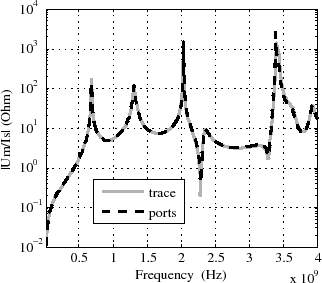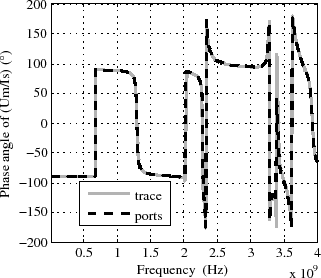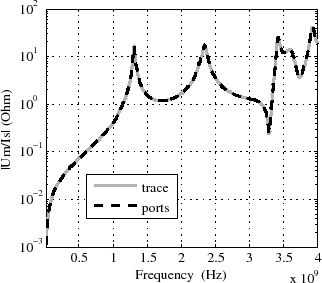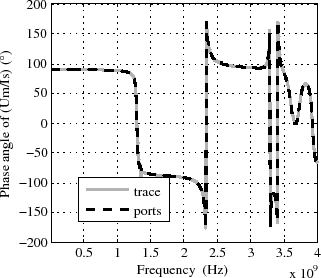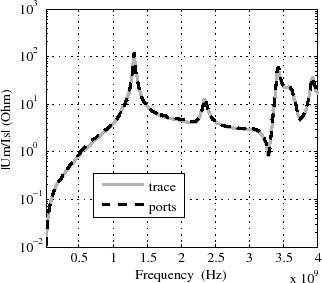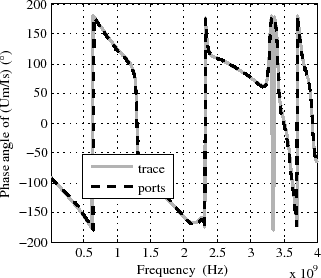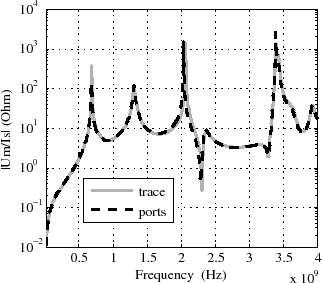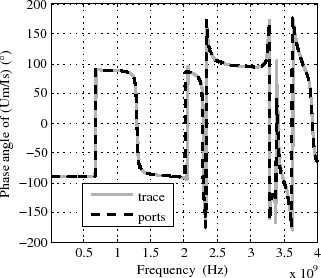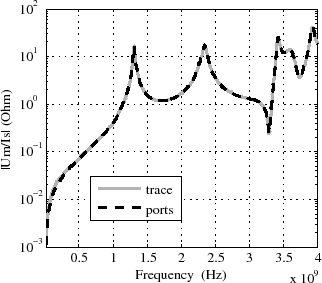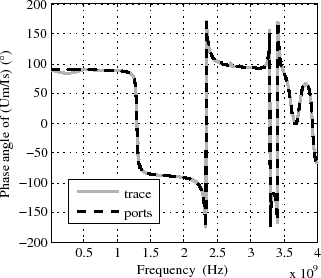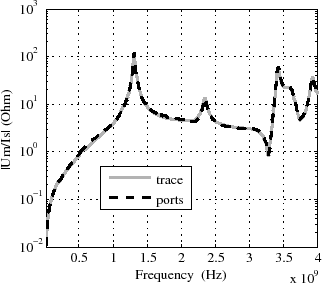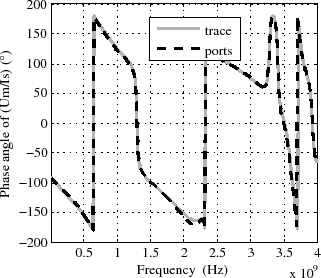# 5.4 Independence of the common mode coupling from the horizontal trace routing

It has previously been mentioned that only the vertical current segments of the trace couple to the cavity. Therefore, the trace introduction method only considers these vertical source and load currents in (5.6). Transfer impedance comparisons as described before are carried out for the two trace routings in Figure 5.7, to validate the independence of the coupling from the horizontal trace segments. The trace length in both models is l=110mm, the trace width=2mm, the trace hight above the ground plane is d=0.65mm, and the enclosure dimensions are (L=134mm, W=104mm, h=7mm). The comparison of the transfer functions to the meas port at (x=67mm, y=104mm) for the models in Figure 5.7(a) and in Figure 5.7(b) are depicted in Figure 5.8 and Figure 5.9 respectively. Comparison results of the transfer impedance to the other two measurement ports are presented in Appendix A.2

Magnitude and phase comparisons show a very good agreement of both models in Figure 5.7(a) and in Figure 5.7(b) to the results obtained with (5.6), (5.9) and the impedance matrix (4.17) from the model in Figure 5.7(c). That confirms the independence of the common mode coupling from the horizontal trace routing and validates the trace introduction method.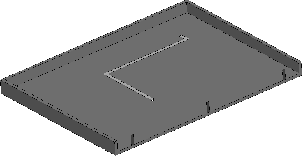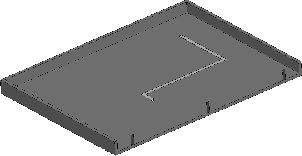(a) Model a with trace. (b) Model with a different trace routing.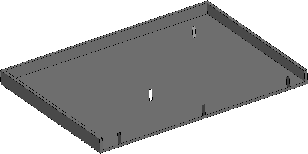(c) Model with ports at the trace source- and load positions.
Figure 5.7: HFSS® models with a different trace routing between identical source and load positions.